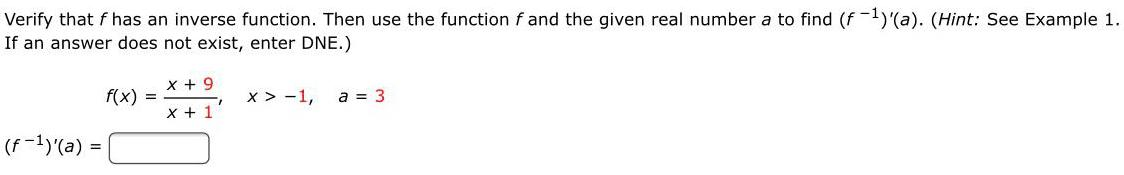Question:

# Verify that f has an inverse function. Then use the function

Last updated: 7/28/2022Verify that f has an inverse function. Then use the function f and the given real number a to find (f-¹)'(a). (Hint: See Example 1. If an answer does not exist, enter DNE.) f(x) =x + 9/x + 1 x > -1, a = 3 (f-¹)'(a)=